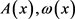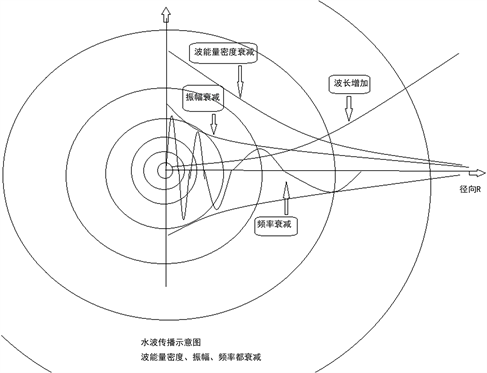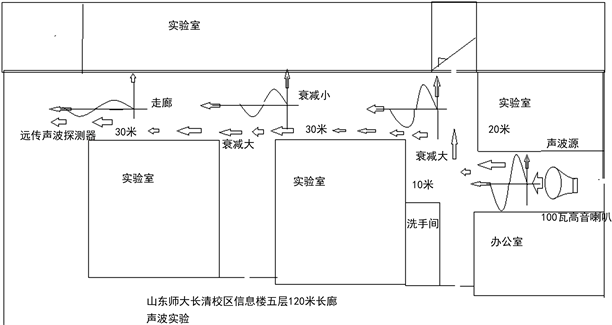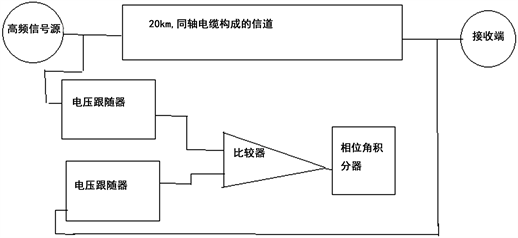# 基于陈寿元效应对宇宙大爆炸论的批判Criticism of the Big Bang Theory Based on Shouyuan Chen Effect

DOI: 10.12677/AAS.2019.73005, PDF, HTML, XML, 下载: 4,135  浏览: 8,454

Abstract: The theory of the Big Bang has been questioning and debating ever since its birth. This paper first introduces Shouyuan Chen’s effect that frequency decreases slowly with propagation distance, then explains the core basis of the Big Bang theory. Practice is the only criterion to test all theories, and criticizes the fallacies of the Big Bang theory from the perspectives of physics, astronomy, philosophy and other disciplines. The Big Bang of the Universe: The Big Bang conclusion of the origin of the whole universe is not the most absurd, only more absurd, than the conclusion of the earth's core that the universe revolves around the earth. The comedy of the Big Bang should come to an end. Only light from distant galaxies measured by Hubble moves toward the red end. As-suming that the red shift is caused by the Doppler effect, it is inferred that the origin of the universe may have come from a big explosion. Shouyuan Chen discovered in 2016 that the frequency of mechanical wave and electromagnetic wave has a very weak attenuation in the process of propagation, which is referred to as the Shouyuan Chen effect. The red shift measured by Hubble is discussed by Shouyuan Chen effect. It is considered that the energy density of light wave decreases due to the ultra-long distance propagation of light wave in space, which leads to the great attenua-tion of light wave amplitude, because the frequency of light wave is also the energy factor of light wave. The attenuation of the energy density of the light wave can also lead to a very slow attenua-tion of the frequency. With Hubble coefficient, light wave propagates in one meter space. The rela-tive variation of frequency attenuation is only 10−26. At present, the accuracy of the international atomic clock is 10−15. Therefore, how to improve the accuracy of measurement becomes the key, starting from the experiment of frequency attenuation of mechanical waves such as water waves and acoustic waves. Ultra-long-distance sensor becomes a key issue. Acoustic wave uses a highly sensitive sensor with built-in two-stage amplifier integrated circuit. At 100 meters, the frequency of 1,000 Hz acoustic wave attenuates by 0.1 Hz. Electromagnetic wave uses 20,000 m, 75-5 coaxial cable, wave source uses 20 MHz high frequency signal, input and output frequency phase angle weak difference for a long time integration, measured the extremely weak attenuation of electro-magnetic wave, electromagnetic wave after 20,000 m propagation, frequency attenuation only 10−6 Hz. Its redshift is about 10−18/m. With the Shouyuan Chen’s effect, the red shift is caused by the exponential attenuation of frequency with the increase of propagation distance caused by the attenuation of wave energy. It can approximate the linear proportional relationship, which is con-sistent with Hubble’s law. The proportional coefficient here has a clear physical meaning. The at-tenuation coefficient of wave frequency with propagation distance is Hubble’s law, which is only called Hubble’s coefficient, but in Hubble’s law, it is not any physical meaning. The probability of cosmic redshift caused by Doppler effect is almost zero, which is a common property of all waves in the process of propagation in a broader sense. The cosmic red shift is attributed to Shouyuan Chen effect, which is more scientific and more in line with modern electromagnetic wave transmission theory and modern communication model. The probability should be 100%. The Doppler effect is roughly the contradiction between the Big Bang theory and other sciences. The universe does not need to explode, and all contradictions are solved. Supernovae discovery: brightness attenuation is greater, redshift is greater; this is inferring the accelerated expansion of the universe. Supernova burst signal action time is very short, which can be seen as a pulse. We received the optical signal, the pulse signal on the transmission channel impulse impact effect, impulse response. Widening the received signal pulse will inevitably result in greater brightness attenuation and redshift. The argument for accelerated expansion of the universe (despite winning the Nobel Prize in physics in 2011) is not sufficient and credible. This paper criticizes the theory of the Big Bang from every scientific point of view. The theory of the Big Bang should be a good hypothesis. World civilization has seriously misled the origin of the universe and had a negative impact. This paper concludes that the red shift of Hubble’s law is caused by the propagation of light wave, not by the backward Doppler effect of galaxies. The Big Bang is only a reasoning hypothesis based on Doppler effect, not true. The Big Bang Reasoning Hypothesis forms a farce that affects all mankind. It's time to call the curtain.

1. 引言

2. 宇宙大爆炸论的天文观测依据

1929年，哈勃利用当时全世界口径最大的光学望远镜(口径：2.54米)，测量到河外遥远星系，发来的光，其谱线有向红的一点移动的现象(就是频率降低)，不认为是一个全新的物理规律。沿用1846年多普勒发现的效应，波源移动，引起波长变化，用其解释测量现象，得到星系后退，后退速度与星系距离成比例的关系式，被称为哈勃定律。

1964年，彭齐亚斯和威尔逊在巨型号角天线上，测量到2.7的微波背景辐射，被定义为是宇宙大爆炸的余辉，获得1978诺贝尔物理奖；1989年11月，约翰·马瑟、乔治·斯穆特主持升空COBE卫星，测量到符合温度为2.726 K的黑体辐射谱的背景辐射，再一次认定为大爆炸余辉，并获得2006诺贝尔物理奖。

1999年，索尔·波尔穆特、布莱恩·施密特和亚当·里斯研究超新星，发现亮度衰减更快、红移更大，推理出宇宙加速膨胀的结论，并获得2011诺贝尔物理奖。

3. 陈寿元效应

3.1. 定义

3.2. 陈寿元效应的理论论述

${\varnothing }_{B}-{\varnothing }_{A}=-E\left(B-A\right)$ (1)

(1)式中：E——能场强度，克服能量耗散而做功。波动从A点传递到B点的必要条件： ${\varnothing }_{A}>{\varnothing }_{B}.$

A点的能场强度 ${E}_{A}$

${E}_{A}=-\underset{B\to A}{\mathrm{lim}}\left(\frac{{\varnothing }_{B}-{\varnothing }_{A}}{B-A}\right)$ (2)

$\varnothing \left(r\right)=\frac{Q}{4\text{ }\in \text{ }\text{π}\left(r-{r}^{\prime }\right)}$ (3)

E——能场强度：可用能位函数的负梯度来表示：

$E=-\nabla \varnothing$ (4)

3.2.1. 质点的无阻尼自由振动

$y=A\mathrm{cos}\left(\omega t+\theta \right)$ (5)

$u=\frac{\text{d}y}{\text{d}t}=-A\omega \mathrm{sin}\left(\omega t+\theta \right)$ (6)

$\alpha =\frac{{\text{d}}^{2}x}{\text{d}{t}^{2}}=-{\omega }^{2}A\mathrm{cos}\left(\omega t+\theta \right)$ (7)

${E}_{k}=\frac{1}{2}m{\upsilon }^{2}=\frac{1}{2}m{A}^{2}{\omega }^{2}{\mathrm{sin}}^{2}\left(\omega t+\theta \right)$ (8)

${\varnothing }_{k}=\frac{{E}_{K}}{m}=\frac{1}{2}{\omega }^{2}{A}^{2}{\mathrm{sin}}^{2}\left(\omega t+\theta \right)$ (9)

(8)和(9)式表明质点的振动能 ${E}_{K}$ 、振动能位函数与振动的频率平方成正比。

3.2.2. 振荡电偶极子产生的电磁波能

$p=gL=gl\mathrm{cos}\omega t$ (10)

${E}_{\theta }=\frac{1}{4\text{π}{\epsilon }_{0}}\frac{{P}_{0}{\omega }^{2}\mathrm{sin}\theta }{{C}^{2}r}\mathrm{cos}\left(\omega t-kr\right)$ (11)

${B}_{\text{op}}=\frac{{\mu }_{0}}{4\text{π}}\frac{{P}_{0}{\omega }^{2}\mathrm{sin}\theta }{Cr}\mathrm{cos}\left(\omega t-kr\right)$ (12)

(11)、(12)式表明，振荡电偶极子在远处产生电场、磁场强度与振荡频率平方成正比。

$S=E×H=\frac{{P}_{0}^{2}{\omega }^{4}{\mathrm{sin}}^{2}\theta }{16{\text{π}}^{2}{\epsilon }_{0}{C}^{3}{r}^{2}}{\left(\mathrm{cos}\left(\omega t-kr\right)\right)}^{2}$ (13)

(13)式表明电偶极子辐射电磁波的能流密度与偶极子振荡频率四次方成正比。

3.2.3. 波动的能位函数

$Y\left(x,t\right)=A\left(x\right)\mathrm{cos}\left(\omega \left(x\right)t-kx\right)$ (14)

(14)式中：A(x)——波的振幅，通常随传播距离而衰减，是X的函数。 $\omega \left(x\right)$ 角速度，目前认为它不随传播距离变化，是不变量。但是前面的分析，振源能量与频率平方成正比。能量是要消耗，扩散、色散。能量在空间上的散开，表现占用更大面积或更大的体积空间，使波长变长。在时域上，能量散开意味着占用更多的时间段，使振动的周期有延长的趋势。

${\upsilon }_{y}=\frac{\partial Y\left(x,t\right)}{\partial t}=-A\left(X\right)\omega \left(X\right)\mathrm{sin}\left(\omega t-kx\right)$ (15)

${\text{op}}_{y}\left(x,t\right)=\frac{1}{2}{\upsilon }_{y}^{2}=\frac{1}{2}{A}^{2}\left(x\right){\omega }^{2}\left(x\right){\left(\mathrm{sin}\left(\omega t-kx\right)\right)}^{2}$ (16)

(16)式表明波动的能位函数与波动的频率平方成正比。

$\text{op}\left(x\right)=\frac{1}{4}{A}^{2}\left(x\right){\omega }^{2}\left(x\right)$ (17)

$\frac{\text{d}p}{\text{d}x}=-\alpha p$

$p\left(x\right)=p\left(0\right){\text{e}}^{-\alpha x}$ (18)

$\frac{\text{dop}}{\text{d}x}=-\alpha \text{op}$

$\text{op}\left(x\right)=\text{op}\left(0\right){\text{e}}^{-\alpha x}$ (19)

${A}^{2}\left(x\right){\omega }^{2}\left(x\right)={A}^{2}\left(0\right){\omega }^{2}\left(0\right){\text{e}}^{-\alpha x}$ (20)

$A\left(x\right)\omega \left(x\right)=A\left(0\right)\omega \left(0\right){\text{e}}^{-\frac{1}{2}\alpha x}$ (21)

1) 若信号传输过程中，频率不变，即：

$\omega \left(x\right)=\omega \left(0\right)$

2) 若信号传输过程中，振幅保持不变，即：

$A\left(x\right)=A\left(0\right)$

3)共同分担信号的衰减量：

$A\left(x\right)=A\left(0\right){\text{e}}^{-\frac{1}{4}\alpha x}$ (24)

$\omega \left(x\right)=\omega \left(0\right){\text{e}}^{-\frac{1}{4}\alpha x}$ (25)

4) $A\left(x\right) (26)

5) $\omega \left(x\right)<\omega \left(0\right){\text{e}}^{-\frac{1}{2}\alpha x}$ (27)

6) 更一般现实情况，A(x)衰减快， $\omega \left(x\right)$ 衰减慢。波的形态分三段：波能量密度很大，随传播距离，振幅衰减为主，频率衰减为辅——称为波状态；波能量密度小，振幅衰减为辅，频率衰减为主——量子状态；波能量密度非常小，振幅、频率都快速衰减为零——波消亡态。从 $k{\text{e}}^{-\frac{1}{2}\alpha x}$${\text{e}}^{-\frac{1}{4}\alpha x}$ 之间变化。

3.3. 实验的技术方案

3.3.1. 水波观察Figure 1. Water wave propagation

3.3.2. 声波实验Figure 2. Acoustic experimental site

3.3.3. 电磁波实验

$z=\frac{\lambda -{\lambda }_{e}}{{\lambda }_{e}}=\frac{{H}_{0}}{c}D$ (28)

$D=2×{10}^{6}×3.086×{10}^{13}\text{km}=6.172×{10}^{22}\text{m}$

${H}_{0}=500\text{\hspace{0.17em}}\text{km}\cdot {\text{s}}^{-1}\cdot {\text{Mpc}}^{-1}$

${H}_{0}=75\text{\hspace{0.17em}}\text{km}\cdot {\text{s}}^{-1}\cdot {\text{Mpc}}^{-1}$ 时，计算每一米的太空频率衰减： $z=0.81×{10}^{-26}$

$\Delta \lambda =\lambda -{\lambda }_{e}=z{\lambda }_{e}=\frac{{H}_{0}}{C}D{\lambda }_{e}$ (29)

$z=\frac{\lambda -{\lambda }_{e}}{{\lambda }_{e}}=\frac{\frac{c}{f}-\frac{c}{{f}_{e}}}{\frac{c}{{f}_{e}}}=\frac{{f}_{e}-f}{f}$

$\Delta f={f}_{e}-f=zf=\frac{{H}_{0}}{c}Df$ (30)

$\Delta \theta =360\left({f}_{e}-f\right)t=360\frac{{H}_{0}}{C}Dft$ (2.31)Figure 3. A schematic diagram of the measurement of weak attenuation of electromagnetic wave frequency

4. 对宇宙大爆炸论讨论

4.1. 宇宙红移的解释

4.2. 超新星的解释

1989年，索尔·波尔穆特、布莱恩·施密特和亚当·里斯研究超新星，发现亮度衰减更快、红移更大，用多普勒效应解释，推理出宇宙加速膨胀的结论，并获得2011诺贝尔物理奖。

4.3. 背景辐射

4.4. 宇宙物质风度

4.5. 宇宙大爆炸与哲学矛盾

4.6. 宇宙大爆炸与物理学矛盾

4.7. 宇宙大爆炸与地心说对比

4.8. 宇宙大爆炸与日心说比较

5. 结论

1) 把宇宙红移现象用多普勒效应来解释，得到宇宙膨胀、宇宙爆炸的结论；用频率衰减来解释，光的红移现象就是光频率在传播过程中，由于波能能量消耗，使频率减小，这将导致波长增长。实验表明，振幅衰减快，频率衰减非常缓慢。在目前技术手段下，难以测量到这种微小变化。通信工程，几万公里的传播范围内，可以认为频率不减小。超精密实验设计可以测量到频率极其微弱的衰减。

2) 宇宙大爆炸论与其他科学矛盾重重，用光信号在太空传播，频率衰减，一切矛盾迎刃而解。

  Yuksel, H., Kistler, M.D., Beacom, J.F. and Hopkins, A.M. (2008) Revealing the High Red-Shift Star Formation Rate with Gam-ma-Ray Bursts. The Astrophysical Journal, 683, L5-L8. https://doi.org/10.1086/591449  Wang, F.Y. and Dai, Z.G. (2009) High Red-Shift Star Formation Rate up to Z~8.3 Derived from Gamma-Ray Bursts and Influence of Background Cosmology. Monthly Notices of Royal Astronomical Society, 400, L10. https://doi.org/10.1111/j.1745-3933.2009.00751.x  Dado, S. and Dar, A. (2014) Long Gamma Ray Bursts Trace the Star Formation History. The Astrophysical Journal, 785, 70.  Wang, F.Y. (2013) The High Red-Shift Star Formation Rate Derived from Gamma-Ray Bursts Origin and Cosmic Reionization. Astronomy & Astrophysics, 556, A90. https://doi.org/10.1051/0004-6361/201321623  Hopkins, A.M. and Bencom, J.F. (2006) On the Normalization of the Cosmic Star Formation History. The Astrophysical Journal, 651, 142-154. https://doi.org/10.1086/506610  陈寿元. 万有阻力定律的几点天文观测验证[J]. 山东师范大学学报, 2000(4): 16.  Sokolov, V.V. (2013) The Gamma-Ray Bursts and Core-Collapse Supernovae-Global Star Forming Rate Peaks at Large Red-Shifts.  Perlmutter, S., et al. (1999) Measurements of Ω and Λ from 42 High-Redshift Supernovae. The Astrophysical Journal, 517, 565-586.  Seeds, M.A. (1998) Astronomy: The Solar System and Beyond. Wadsworth Publishing Company, Belmont, 355-356.  李宗伟, 肖兴华. 天体物理学[M]. 北京: 高等教育出版社, 2000: 426-472.  陈寿元. 信息物理[M]. 济南: 山东地图出版社, 2008: 5.  凯德洛夫, 等. 物理学的方法论原理[M]. 柳树滋, 等, 译. 北京: 知识出版社, 1990: 447.  [美] Hewitt, P.G. (1989) Conceptual Physics. Mc-Graw Hill, New York.  程守洙, 江之水. 普通物理学[M]. 北京: 高等教育出版社, 1961.  [美] Guruan, B. (2005) Electromagnetic Field Theory Fundamentals. Second Edition, China Machine Press, Beijing, 1.  蔡圣善, 等. 电动力学[M]. 第二版, 北京: 高等教育出版社, 2002.  贾起民, 等. 电磁学[M]. 第二版, 北京: 高等教育出版社, 2001.  王家礼, 朱满堂, 著. 电磁场与电磁波[M]. 西安: 西安电子科技大学出版社, 2009.  Communications Week, 1993, 9.  Rappaport, T.S. (1996) Wireless Communications Principles & Practice. Prentice Hall, Upper Saddle River.  郭梯云, 邬国杨, 李建东. 移动通信[M]. 西安: 西安电子科技大学出版社, 2001.  李兆玉, 何维, 戴翠琴. 移动通信[M]. 北京: 电子工业出版社, 2017.  陈显治, 等. 现代通信技术[M]. 北京: 电子工业出版社, 2004.  赵洪波, 卜益民, 陈风娟. 现代通信技术概论[M]. 北京: 北京邮电大学出版社, 2003.  张传福, 彭灿, 胡熬, 刘晓甲, 卢辉斌. CDAM 移动通信网络规划设计与优化[M]. 北京: 人民邮电出版社, 2006.  苏华鸿, 孙儒石, 等. 蜂窝移动通信射频工程[M]. 北京: 人民邮电出版社, 2005.  冯玉珉. 通信系统原理[M]. 北京: 清华大学出版社, 2003.  Tabbans, S. 无线移动通信网络[M]. 李新付, 等, 译. 北京: 电子工业出版社, 2001.  孙宇彤, 等. CDMA空中接口技术[M]. 北京: 人民邮电出版社, 2004.  Rhee, M.Y. (2002) CDMA Cellular Mobile Communications and Network Security. Prentice Hall, Upper Saddle River.  Feher, K. (1995) Wireless Digital Communication. Prentice Hall, Upper Saddle River.  GSM Recommendation 03.01, ETS300 521-1994, 1994, 1.  牛顿. 自然哲学之数学原理[M]. 王克迪, 译. 北京: 北京大学出版社, 2006.  Seeds, M.A. (1998) Astronomy: The Solar System and Beyond. Wadsworth Publishing Company, Belmont.  庄德新, 聂清香. 天文学[M]. 济南: 山东大学出版社, 2002.  伽利略. 关于托勒密和哥白尼两大世界体系的对话[M]. 周熙良, 等, 译. 北京: 北京大学出版社, 2006.  戴文赛. 太阳系演化学[M]. 北京: 科学出版社, 1980.  哥白尼. 天体运行论[M]. 叶式辉, 译. 北京: 北京大学出版社, 2006.  李宗伟, 肖兴华. 天体物理学[M]. 北京: 高等教育出版社, 2000.  陈寿元. 相对静止轮[J]. 山东大学学报(工学版), 2002, 32(4): 396-400.  Dampier, W.C. 科学史及其哲学与宗教的关系[M]. 李珩, 译. 桂林: 广西师范大学出版社, 2001: 105-110.  杜功焕, 等. 声学基础[M]. 第二版, 南京: 南京大学出版社, 2001.  曽心传, 等. 震动理论基础[M]. 北京: 地震出版社, 2013.  易克初, 等. 语音信号处理[M]. 北京: 国防工业出版社, 2000.  陈熙谋. 光学, 近代物理学[M]. 北京: 北京大学出版社, 2002.  刘增基, 等. 光纤通信[M]. 西安: 西安电子科技大学出版社, 2001.  易搏藤, 等. 电磁学简明教程[M]. 北京: 北京师范大学出版社, 2001.  约翰克劳斯. 天线[M]. 章文勋, 译. 北京: 电子工业出版社, 2004.  陈寿元. 相对静止论[J]. 山东大学学报(工学版), 2002, 32(4): 396-400.  陈宇, 陈寿元. 基于现代通信模型的遥远星系光色变化的研究[J]. 山东师范大学学报(自然科学版), 2019, 34(1): 61-69.  陈宇, 陈寿元. 天文信息处理模式研究[J]. 天线学报, 2018, 7(4): 25-34.  陈寿元. 频率衰减: 哈勃红移——陈寿元效应[J]. 科技信息, 2017(11): 50.  陈宇, 陈寿元. 信道天文学[简介]: 通信模式处理天文信号[J]. 天文宇天体物理, 2019(1): 21-30.  陈寿元. 基于陈寿元效应的水波频率衰减、红移实验装置及方法[P]. 受理号: 2017104325114.  陈寿元. 基于陈寿元效应的声波频率衰减、红移实验装置及方法[P]. 受理号: 2017104325006.  陈寿元. 基于陈寿元效应的电磁波频率衰减、红移实验装置及方法[P]. 受理号: 201710432711X.  陈寿元. 基于陈寿元效应的微波频率衰减、红移实验装置及方法[P]. 受理号: 2017104326988.  陈寿元, 陈宇. 一种基于陈寿元效应的电磁波红移测量系统及方法[P]. 受理号: 2017104538500.  陈寿元, 陈宇. 一种基于陈寿元效应的声波红移测量系统及方法[P]. 受理号: 201710454768X.  陈寿元, 陈宇. 一种基于陈寿元效应的水波红移测量系统及方法[P]. 受理号: 2017104537851.  陈寿元, 孙跃鲁, 李文健.,远距离通信系统与方法[P]. 受理号: 2017104517858.  陈寿元, 陈宇. 一种基于光波红移的天文观测望远镜[P]. 受理号: 2017104526749.  戴文赛. 太阳系演化学[M]. 北京: 科学出版社, 1980.  李艳平, 申先甲. 物理学史教程[M]. 北京: 科学出版社, 2003: 102.  凯德洛夫, 等. 物理学的方法论原理[M]. 柳树滋, 等, 译. 北京: 知识出版社, 1990.  关士续, 等. 自然辩证法概论[M]. 北京: 高等教育出版社, 2002: 49-52.  孙学康, 张金菊. 光纤通信技术[M]. 北京: 北京邮电大学出版社, 2001.